Wednesday, 26 December 2012

CBSE Class 9 - Science - CH 4 - STRUCTURE OF THE ATOM

STRUCTURE OF THE ATOMBohr Model

NCERT Solutions, Q & A

Q1(CBSE 2011): Name the fundamental particle not present in the nucleus of hydrogen atom

Q2: Who discovered electrons?

Q3: Who discovered protons?

Q4(CBSE 2011): Who discovered neutrons?

Q5: Who discovered nucleus of an atom?

Q6(NCERT): What are canal rays?

1. Canal rays are positively charged radiations due to positive charged sub-atomic particles.
2. These are also called anode rays.
3. These were discovered by E. Goldstein in 1866.
4. Canal rays pass through perforated cathode and then travel towards another cathode in a gas discharge tube.

Q7: What are the properties of anode rays?

1. Anode rays travel along straight path and cast shadow of the objects placed in their path.
2. They rotate a light paddle wheel placed in their path. It indicates the anode rays consist of particles and carry energy.
3. They deflect towards negatively charged plate. It shows anode rays carry positive charge.

Q8: What are cathode rays?

Answer: Cathode rays are high speed beam of electrons emitted from cathode and possess negative charge. Cathode rays were discovered by J. J. Thomson and Crookes experimentally. Cathode rays travel in straight line and are electromagnetic in nature.

Watch the you tube video on cathode rays:

Q9: Who discovered X-Rays?

Answer: Rontgen in 1896 (Also called Rontgen rays).

Q10(NCERT): If an atom contains one electron and one proton, will it carry any charge or not?

Answer:  No atom will not carry any charge and remains neutral. The unit negative charge on an electron will cancel out the effect of unit positive charge on the proton. Thus the net charge on the atom is zero.

Q11: Complete the following table.

ParticleElectronProtonNeutron
(i)   Symbol__ __ __
(ii)  Nature__ __ __
(iii) Relative Charge__ __ __
(iv) Absolute Charge__ __ __
(v)  Relative Mass__ __ __
(vi) Absolute Mass__ __ __

ParticleElectronProtonNeutron
(i)   Symbol   e-   p+   n
(ii)  Nature  negatively charged    Positively Charged    Neutral
(iii) Relative Charge  -1   +1   0
(iv) Absolute Charge  1.602 × 10-19 C   1.602 × 10-19 C   0
(v)  Relative Mass  1/1840   1   1
(vi) Absolute Mass  9.109 × 10-28 g   1.67 ×10-24g  1.67 × 10-24 g

Q12(CBSE 2011): What is the mass of proton as compared to electron?

Answer: Mass of proton was found approximately 2000 times (1840 times) as that of electron. It is 1.67 ×10-24g approximately.

Q13(CBSE 2011): Describe briefly Thomson's model of an atom.Thomson's Model
Answer: Thomson proposed the model of an atom to be similar to that of a Christmas pudding. The electrons, in a sphere of positive charge, were like currants (dry fruits) in a spherical Christmas pudding.

• An atom consists of a positively charged uniform sphere and the electrons are embedded in it.
• The negative and positive charges are equal in magnitude. So, the atom as a whole is electrically neutral.
• Mass of the atom is considered to be uniformly distributed.

Q14(CBSE 2011): Write the limitations of J.J. Thomson's model of an atom.

Answer: Although Thomson's model of atom was able to explain electrical neutrality of the atom. However it failed to explain how these fundamental particles (electrons and positive charges) were arranged in the atom. Neither it explained how come free positive and negative charges came too close to each other.

Q15: What are α-particles?

Answer: α-particles are doubly-charged helium ions with 4 units of mass. These are produced from radioactive sources like Radium or Polonium.
42He → He2+ + 2e-

Q16(NCERT): On the basis of Rutherford’s model of an atom, which subatomic particle is present in the nucleus of an atom?

Answer: On the basis of Rutherford’s model of an atom, there is a positively charged centre in
an atom called the nucleus. It indicates protons are present in the nucleus of the atom.

Q17: Who is known as 'Father of Nucleus Physics'?

See the you tube video on Rutherford's scattering experiment

Q18:  What were the observations of Rutherford's α-scattering experiment?

1. Most of the α particles passed straight through the gold foil without any deflection.
2. Some of the α-particles were deflected by the foil by small angles.
3. A very small percentage ( 1 out of 12000) rebound i.e. deflected by wide angles between 90 - 180.

Q19: What were the important features of atomic model based on Rutherford's scattering experiment?

Answer: Main features of atomic model based on Rutherford's scattering experiment:
1. Most of the space in the atom is empty.
2. Atom consists of a dense small positively charged nucleus which is at the centre of the atom.
3. Electrons are distributed in the empty space around the nucleus and move in concentric orbits.
4. Volume of nucleus is negligibly small as compared to volume of the atom.
5. Charge on Number of electrons is same the total positive charge in the nucleus. Hence atom is electrically neutral.

Q20: What are the limitations of Rutherford’s model of the atom?

1. According to Rutherford's model, electrons revolve around positive charged nucleus. Any particle in circular motion would undergo acceleration. But according to electromagnetic theory, if a charged body (electron) rotates around another charge body (nucleus), it will radiate energy. Due to radiation, loss of energy takes place and speed of electron will decrease. Eventually it will fall into the nucleus. But such collapse does not occur.
2. Rutherford could not explain how many electrons rotate in a particular orbit.

Q21(NCERT): Draw a sketch of Bohr’s model of an atom with three shells.Neil Bohr Atomic Model (Energy shells are also marked as K, L, M...)

Q22(NCERT): Describe Bohr’s model of the atom.

Answer: In 1913, Neil Bohr overcame the limitations of Rutherford model and proposed a model model of atomic structure. It has the following postulates:

1. Electrons revolve around the nucleus in a limited number of orbits called permissible or discrete orbits.
2. These discrete orbits have definite energy hence they are called energy levels or energy shells. These orbits or shells are represented by the letters K,L,M,N,… or the numbers, n=1,2,3,4,…
3. The energy of an electron remains constant so long as it stays in the given energy level. It means electrons present in different orbits have different energies.
4. When an electron jumps from lower energy level to higher energy level, some energy is absorbed. While some amount of energy is released when an electron jumps from higher energy level to lower one.
Q 23(NCERT): What do you think would be the observation if the α-particle scattering experiment is carried out using a foil of a metal other than gold?

Answer: Gold foil was chosen because gold is malleable and the foil can be made as thick as 1000 atoms. Foils made by other metals if are thicker than the gold foil, the scattering result may not be exactly the same. It may be due to absorption of electrons by thicker foil and we could not reach the same inference. If the foil is as thick as the gold foil, inference would be the same.

Q 24: Write a short note on Nucleus.

1. Nucleus of an atom consists of fundamental particles, protons and neutrons, collectively called as nucleons.
2. Nucleus is positively charged due to the presence of protons.
3. Nucleus is highly dense and largely reponsible for the mass of the atom.
4. Size of the nucleus as compared to atomic size is very less (1000th of atomic radius).

Q 25: What is Atomic Number? Who coined this term?

Answer: The number of protons present inside an atom is equal to its atomic number. It is denoted by symbol Z. Since atom is electrically neutral due to presence of electrons.

Atomic Number (Z) = No. of Protons = Number of Electrons

Mosely (1913) proposed the total positive charge on an atom is equal to its atomic number.

Q 26: What is mass number?

Answer: Mass number (A) is equal to number of nucleons present inside the nucleus. It
means it is the sum of number of protons and neutrons present in the nucleus of an atom. It is
denoted by letter A.

Q 27: If A = 23 and Z = 11 for Na atom, how many protons, electrons and neutrons present in Na atom?

Answer: A = 23, Z = 11
No. of e- = No. or protons = Z
Number of electrons outside nuclues = 11
Number of electrons inside nuclues = 11
No. of neutrons = A - Z = 23 -11 = 12

Q28: What is ionization energy?

Answer: When an atom loses one electron, it becomes a positive ion. Energy required to remove an electron from each atom of an element is called ionization energy.

(In progress...)1.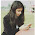2.thanks.................

3.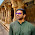4.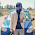very useful for exams

5.very useful for exams

6.useful for exams

7.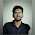Who discovered proton .
Goldstein or Rutherford.

1.2.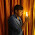it is E.goldstien who discovered proton,ghanashyam shekhar

3.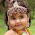This comment has been removed by the author.

4.Nice questions really helpful in exam... Thank you so much

5.8.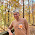why cant i print this or copy it

9.10.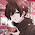very very cool

11.1.2.This comment has been removed by the author.

12.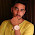13.This comment has been removed by the author.

14.15.16.1.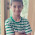2.17.This comment has been removed by the author.

18.simply than simply yo keep lolling

19.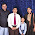ya bro simply lolling

20.This comment has been removed by the author.

21.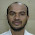Need competitive level questions set...though work done is good...

22.1.2.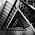23.24.25.It's useful 😀😀😀😀😀😀😀😀💂

26.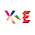This comment has been removed by the author.

27.